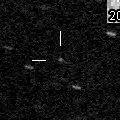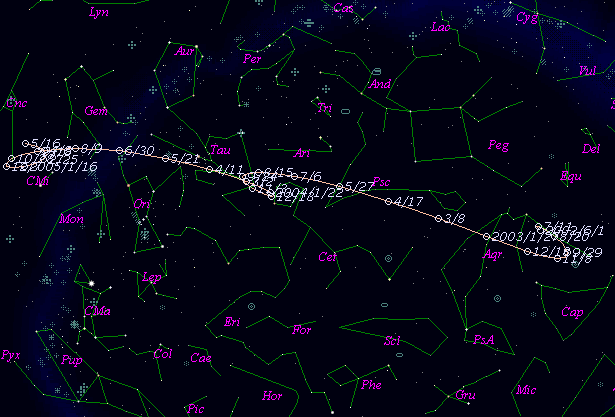# \$B%[%\$%C%W%kWB@1(B

36P/Whipple (2003)###\$B###\$B50F;MWAG(B

```   The following improved orbital elements by Kenji Muraoka, are
from 220 observations  1933 to 2000, including 9 Planets, Moon
and 5 minor planets perturbations and non-gravitational effect
of style II.     The mean residual is +/- 0.91 arc seconds.

Epoch  =  2003 July 20.0  TT       JDT = 2452840.5
T  =  2003 July  6.65184       +/- 0.00065 (m.e.) TT
Peri. =  202.21301                +/- 0.00016
Node  =  182.39909                +/- 0.00010   (2000.0)
Incl. =    9.93291                +/- 0.00002
q  =    3.0882616              +/- 0.0000006 AU
e  =    0.2590415              +/- 0.0000001
a  =    4.1679279              +/- 0.0000003 AU
n  =    0.11583073             +/- 0.00000001
P  =    8.509                  +/- 0.0000008  years
A1  =   +0.343                  +/- 0.017
A2  =   -0.05246                +/- 0.00045
```

###\$B@1?^(B###\$B8wEYJQ2=(B

```        m1 = 8.0 + 5 log\$B&\$(B + 17.0 log r
```##### \$B50F;MWAG\$OB<2,7r<#;a\$N7W;;\$K\$h\$k\$b\$N\$G\$9!#(B \$B@1?^\$O(B StellaNavigator Ver.2.0 for Windows (\$B%"%9%H%m%"!<%D(B \$BJTCx(B / \$B%"%9%-!<=PHG6I4)(B) \$B\$G:n@.\$7\$?\$b\$N\$G\$9!#(B \$B8wEY%0%i%U\$O(BComet for Windows\$B\$G:n@.\$7\$?\$b\$N\$G\$9!#(B